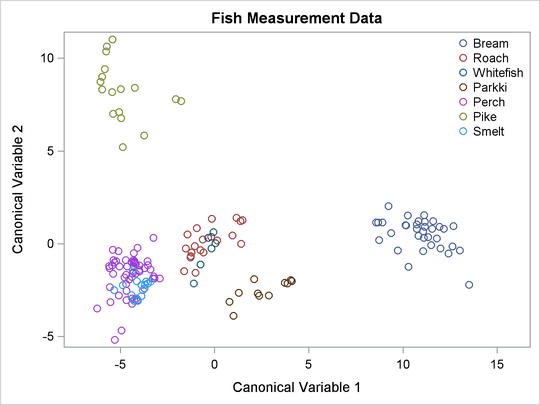# The CANDISC Procedure

## Getting Started: CANDISC Procedure

The data in this example are measurements of 159 fish caught in Finland’s lake Laengelmavesi; this data set is available from the Puranen. For each of the For each of the seven species (bream, roach, whitefish, parkki, perch, pike, and smelt) the weight, length, height, and width of each fish are tallied. Three different length measurements are recorded: from the nose of the fish to the beginning of its tail, from the nose to the notch of its tail, and from the nose to the end of its tail. The height and width are recorded as percentages of the third length variable. The fish data set is available from the Sashelp library.

The following step uses PROC CANDISC to find the three canonical variables that best separate the species of fish in the Sashelp.Fish data and create the output data set outcan. With the NCAN=3 option, only the first three canonical variables are displayed. The ODS EXCLUDE statement is specified to exclude the canonical structure tables and most of the canonical coefficient tables in order to obtain a more compact set of results. The TEMPLATE and SGRENDER procedures are used to create a plot of the first two canonical variables. The following statements produce Figure 29.1 through Figure 29.6:

title 'Fish Measurement Data';

proc candisc data=sashelp.fish ncan=3 out=outcan;
ods exclude tstruc bstruc pstruc tcoef pcoef;
class Species;
var Weight Length1 Length2 Length3 Height Width;
run;

proc template;
define statgraph scatter;
begingraph;
entrytitle 'Fish Measurement Data';
layout overlayequated / equatetype=fit
xaxisopts=(label='Canonical Variable 1')
yaxisopts=(label='Canonical Variable 2');
scatterplot x=Can1 y=Can2 / group=species name='fish';
layout gridded / autoalign=(topright);
discretelegend 'fish' / border=false opaque=false;
endlayout;
endlayout;
endgraph;
end;
run;

proc sgrender data=outcan template=scatter;
run;


PROC CANDISC begins by displaying summary information about the variables in the analysis. This information includes the number of observations, the number of quantitative variables in the analysis (specified with the VAR statement), and the number of classes in the classification variable (specified with the CLASS statement). The frequency of each class is also displayed.

Figure 29.1: Summary Information

 Fish Measurement Data

The CANDISC Procedure

 Total Sample Size DF Total 158 157 6 151 7 6

 Number of Observations Read 159 158

Class Level Information
Species Variable
Name
Frequency Weight Proportion
Bream Bream 34 34.0000 0.215190
Parkki Parkki 11 11.0000 0.069620
Perch Perch 56 56.0000 0.354430
Pike Pike 17 17.0000 0.107595
Roach Roach 20 20.0000 0.126582
Smelt Smelt 14 14.0000 0.088608
Whitefish Whitefish 6 6.0000 0.037975

PROC CANDISC performs a multivariate one-way analysis of variance (one-way MANOVA) and provides four multivariate tests of the hypothesis that the class mean vectors are equal. These tests, shown in Figure 29.2, indicate that not all of the mean vectors are equal (p < .0001).

Figure 29.2: MANOVA and Multivariate Tests

 Fish Measurement Data

The CANDISC Procedure

Multivariate Statistics and F Approximations
S=6 M=-0.5 N=72
Statistic Value F Value Num DF Den DF Pr > F
Wilks' Lambda 0.00036325 90.71 36 643.89 <.0001
Pillai's Trace 3.10465132 26.99 36 906 <.0001
Hotelling-Lawley Trace 52.05799676 209.24 36 413.64 <.0001
Roy's Greatest Root 39.13499776 984.90 6 151 <.0001

The first canonical correlation is the greatest possible multiple correlation with the classes that can be achieved by using a linear combination of the quantitative variables. The first canonical correlation, displayed in Figure 29.3, is 0.987463. A likelihood ratio test is displayed of the hypothesis that the current canonical correlation and all smaller ones are zero. The first line is equivalent to Wilks’ lambda multivariate test.

Figure 29.3: Canonical Correlations

 Fish Measurement Data

The CANDISC Procedure

Canonical
Correlation
Canonical
Correlation
Approximate
Standard
Error
Squared
Canonical
Correlation
Eigenvalues of Inv(E)*H
= CanRsq/(1-CanRsq)
Test of H0: The canonical correlations in the current row and all that follow are zero
Eigenvalue Difference Proportion Cumulative Likelihood
Ratio
Approximate
F Value
Num DF Den DF Pr > F
1 0.987463 0.986671 0.001989 0.975084 39.1350 29.3859 0.7518 0.7518 0.00036325 90.71 36 643.89 <.0001
2 0.952349 0.950095 0.007425 0.906969 9.7491 7.3786 0.1873 0.9390 0.01457896 46.46 25 547.58 <.0001
3 0.838637 0.832518 0.023678 0.703313 2.3706 1.7016 0.0455 0.9846 0.15671134 23.61 16 452.79 <.0001
4 0.633094 0.623649 0.047821 0.400809 0.6689 0.5346 0.0128 0.9974 0.52820347 12.09 9 362.78 <.0001
5 0.344157 0.334170 0.070356 0.118444 0.1344 0.1343 0.0026 1.0000 0.88152702 4.88 4 300 0.0008
6 0.005701 . 0.079806 0.000033 0.0000   0.0000 1.0000 0.99996749 0.00 1 151 0.9442

The first canonical variable, Can1, shows that the linear combination of the centered variables Can1 =–0.0006Weight – 0.33Length1 2.49Length2 + 2.60Length3 + 1.12Height – 1.45Width separates the species most effectively (see Figure 29.4).

Figure 29.4: Raw Canonical Coefficients

 Fish Measurement Data

The CANDISC Procedure

Raw Canonical Coefficients
Variable Can1 Can2 Can3
Weight -0.000648508 -0.005231659 -0.005596192
Length1 -0.329435762 -0.626598051 -2.934324102
Length2 -2.486133674 -0.690253987 4.045038893
Length3 2.595648437 1.803175454 -1.139264914
Height 1.121983854 -0.714749340 0.283202557
Width -1.446386704 -0.907025481 0.741486686

PROC CANDISC computes the means of the canonical variables for each class. The first canonical variable is the linear combination of the variables Weight, Length1, Length2, Length3, Height, and Width that provides the greatest difference (in terms of a univariate F test) between the class means. The second canonical variable provides the greatest difference between class means while being uncorrelated with the first canonical variable.

Figure 29.5: Class Means for Canonical Variables

Class Means on Canonical Variables
Species Can1 Can2 Can3
Bream 10.94142464 0.52078394 0.23496708
Parkki 2.58903743 -2.54722416 -0.49326158
Perch -4.47181389 -1.70822715 1.29281314
Pike -4.89689441 8.22140791 -0.16469132
Roach -0.35837149 0.08733611 -1.10056438
Smelt -4.09136653 -2.35805841 -4.03836098
Whitefish -0.39541755 -0.42071778 1.06459242

A plot of the first two canonical variables (Figure 29.6) shows that Can1 discriminates between three groups: 1) bream; 2) whitefish, roach, and parkki; and 3) smelt, pike, and perch. Can2 best discriminates between pike and the other species.

Figure 29.6: Plot of First Two Canonical Variables